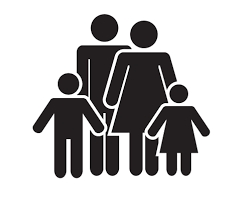Grandma

We're going to Grandma's. We still have 24 km left. How far does Grandma live if we have traveled from the total distance: half, a third, a fifth?

a =  48 km
b =  36 km
c =  30 km

Step-by-step explanation:Did you find an error or inaccuracy? Feel free to write us. Thank you!Tips to related online calculators
Need help to calculate sum, simplify or multiply fractions? Try our fraction calculator.
Do you have a linear equation or system of equations and looking for its solution? Or do you have a quadratic equation?
Do you want to convert length units?